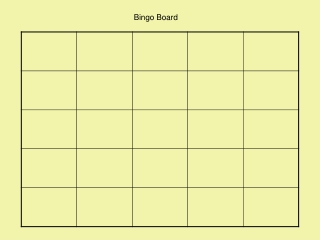DownloadDownload PresentationBingo Board

# Bingo Board

Télécharger la présentation## Bingo Board

- - - - - - - - - - - - - - - - - - - - - - - - - - - E N D - - - - - - - - - - - - - - - - - - - - - - - - - - -
##### Presentation Transcript

1. Bingo Board

2. Standard: Measurement Benchmark: C: Identify appropriate tools for measuring angles, perimeter or circumference and area of triangles, quadrilaterals, circles and composite shapes and surface area and volume of prisms and cylinders. D: Select a tool and measure accurately to a specified level of precision. (5.2.7) Standard: Geometry and Spatial Sense Benchmark: A: Identify and label angle parts and the regions defined within the plane where the angle resides. (5.3.3) D: Identify, describe and classify types of line pairs, angles, two-dimensional figures and three-dimensional objects using their properties. (6.3.1) Standard: Mathematical Processes Benchmark: K: Recognize and use mathematical language and symbols when reading, writing and conversing with others. (6.3.2) (5.3.2)

3. OBJECTIVE: Students can review geometry vocabulary necessary to meet the Ohio State Standards for Measurement, Geometry and Spatial Sense and Mathematical Processes. • EQUIPMENT: Computer, Projector, Print out copies of the Bingo Board • RULES: • Played like regular bingo, except the squares are filled in with Math vocabulary. • Students view slide 2 to choose 25 vocabulary they put randomly on their boards. • Begin the slide show, manually advancing slides based upon the audience of students. • When a student Bingos, they read their words back to the instructor/host. • Note: some answers are better than others for the slides. • When a student Bingos, they create a new board while other students continue using the board they began. • You can shuffle slides each time before you play!!

4. Welcome To Geometry BINGO!

5. Vocabulary Words • Symmetry • Supplementary • Complementary • Side • Degrees • Straight • Angles • Perpendicular • Congruent • Bisects • Polygon • Trapezoid • Similar • Reflection • Rotation • Three • Four • Parallel • Pentagon • Radius • Diameter • Parallelogram • Equilateral • Quadrilateral • Protractor • Compass • 90 • Acute • Obtuse • Right • 180 • 360 • Centimeters • Inches • Segment • Vertex

6. What is this tool?

7. This shape is a ____

8. Name the shape

9. Describe these lines

10. This shape is a ____.

11. What is this tool?

12. What type of triangle is this?

13. This is what type of angle?

14. “B” is the ______. H B J

15. What is the term for this line?

16. The segment is about 7 _____.

17. R This is a ______ angle. M Q

18. What is the term for this line?

19. This part of an angle.

20. How many lines of symmetry?

21. What is this type of angle?

22. How many lines of symmetry for this shape?

23. These numbers measure ___.

24. This transformation is a ___.

25. Line MN _____ line GF. M F G N

26. This type of transformation is a __.

27. This is a line _____. W____________________V

28. These shapes are ____.

29. This is a ____ angle.

30. This angle measures ____.

31. This angle measures ____.

32. This segment is about 3 ____?

33. These are all ___.

34. This shape has a line of ____.

35. The arrows indicate _____ degrees.

36. This figure is a ____.

37. These line segments are __. M W C H

38. These figures are____.

39. These angles are ___. A T 2 1 K B F

40. Name this shape.